# AP Physics 2 Practice Test 16

### Test Information10 questions18 minutes

1.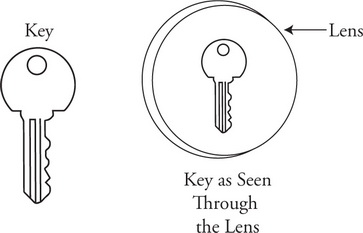A student looks at a key through a lens. When the lens is 10 cm from the key, what the student sees through the lens is shown in the figure. The student estimates that the image is about half the size of the actual key. What is the approximate focal length of the lens being used by the student?

2. A neutron is shot into a uranium atom, producing a nuclear reaction: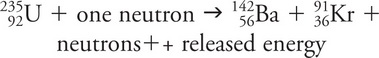Which of the following best describes this reaction?

3.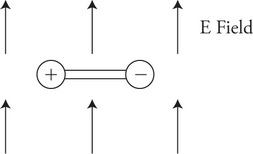Two opposite charges of equal magnitude are connected to each other by an insulated bar and placed in a uniform electric field as shown in the figure. Assuming the object is free to move, how will the object move and why?

4. Which of the following best represents the isolines of electric potential surrounding two identical positively charged spheres?

5. The diagram shows a circuit that contains a battery with a potential difference of VB and negligible internal resistance; five resistors of identical resistance; three ammeters A1, A2, A3; and a voltmeter.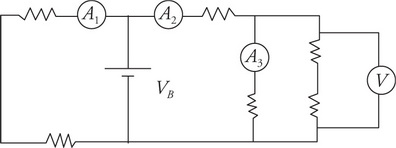Which of the following correctly ranks the readings of the ammeters?

6. The diagram shows a circuit that contains a battery with a potential difference of VB and negligible internal resistance; five resistors of identical resistance; three ammeters A1, A2, A3; and a voltmeter.What will be the reading of the voltmeter?

7.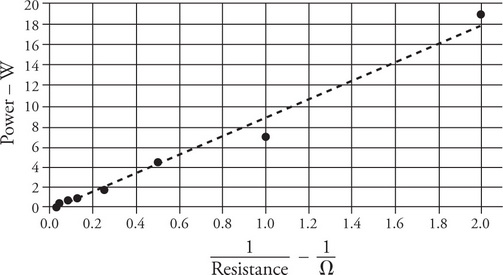A battery of unknown potential difference is connected to a single resistor. The power dissipated in the resistor is calculated and recorded. The process is repeated for eight resistors. A plot of the data with a best-fit line was made and is displayed in the figure. The potential difference provided by the battery is most nearly:

8.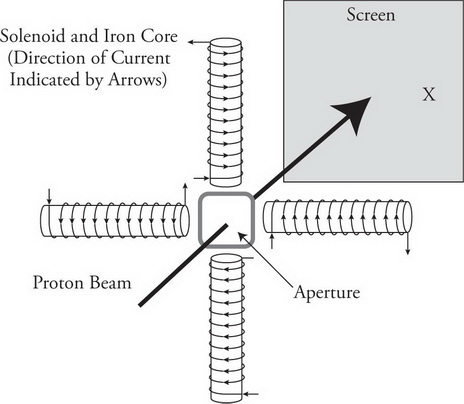A high-energy proton beam is used in hospitals to treat cancer patients. The beam is shot through a small aperture surrounded by four solenoids with iron cores that are used to direct the beam at cancer cells to kill them. (The direction of the current around the solenoids is indicated by the arrows.) During routine maintenance, technicians calibrate the machine by pointing the beam at the center of a screen and then directing it toward designated target points. Which of the four solenoids will the technician use to direct the beam toward the target “X” on the right side of the screen?

9.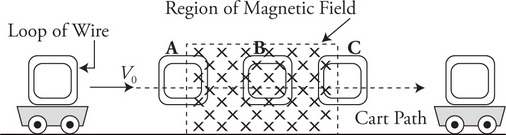A lab cart with a rectangular loop of metal wire fixed to its top, travels along a frictionless horizontal track as shown. While traveling to the right, the cart encounters a region of space with a strong magnetic field directed into the page. The cart travels through locations A, B, and C on its way to the right as shown in the figure. Which of the following best describes any current that is induced in the loop or wire?

10.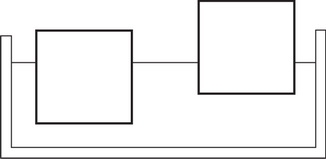Two blocks of the same size are floating in a container of water as shown in the figure. Which of the following is a correct statement about the two blocks?